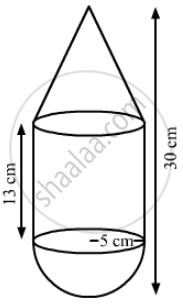Advertisement Remove all ads

# A Toy is in the Shape of a Right Circular Cylinder with a Hemisphere on One End and a Cone on the Other. the Radius and Height of the Cylindrical Part Are 5 Cm and 13 Cm, - Mathematics

Sum

A toy is in the shape of a right circular cylinder with a hemisphere on one end and a cone on the other. The radius and height of the cylindrical part are 5 cm and 13 cm, respectively. The radii of the hemispherical and the conical parts are the same as that of the cylindrical part. Find the surface area of the toy, if the total height of the toy is 30 cm.

Advertisement Remove all ads

#### SolutionWe have,

the base radius of none = the base radius of cylinder = the base radius of hemisphe = r= 5 cm,

the total height of the toy = 30 cm

Also, the height of the cone, h=30 - (13 + 5) = 12 cm

The slant height of the cone, l = sqrt(r^2+ h^2)

= sqrt(5^2 + 12^2)

= sqrt(25+144)

=sqrt(169)

= 13 cm

Now, the surface area of the toy= CSA of cone +CSA of cylinder + CSA of hemisphere

= pirl + 2pirH + 2pir^2
=pirl (l + 2H+2r)

= 22/7xx5xx(13+2xx13+2xx5)

= 22/7xx5xx(13xx26+10)

= 22/7xx5xx49

=770 cm2

So, the surface area of the toy is 770 cm2.

Is there an error in this question or solution?
Advertisement Remove all ads

#### APPEARS IN

RS Aggarwal Secondary School Class 10 Maths
Chapter 19 Volume and Surface Area of Solids
Exercise 19A | Q 30 | Page 877
Advertisement Remove all ads

#### Video TutorialsVIEW ALL 

Advertisement Remove all ads
Share
Notifications

View all notifications

Forgot password?
Course